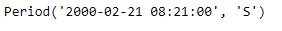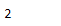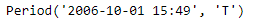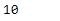# Python | Pandas Period.month

• Last Updated : 06 Jan, 2019

Python is a great language for doing data analysis, primarily because of the fantastic ecosystem of data-centric python packages. Pandas is one of those packages and makes importing and analyzing data much easier.

Pandas` Period.month` attribute return an integer value. The returned value represents the value of month in the given period object. January being 1 to December being 12.

Attention geek! Strengthen your foundations with the Python Programming Foundation Course and learn the basics.

To begin with, your interview preparations Enhance your Data Structures concepts with the Python DS Course. And to begin with your Machine Learning Journey, join the Machine Learning - Basic Level Course

Syntax : Period.month

Parameters : None

Return : month

Example #1: Use `Period.month `attribute to find the value of month in the given Period object.

 `# importing pandas as pd``import` `pandas as pd`` ` `# Create the Period object``prd ``=` `pd.Period(freq ``=``'S'``, year ``=` `2000``, month ``=` `2``,``                 ``day ``=` `21``, hour ``=` `8``, minute ``=` `21``)`` ` `# Print the Period object``print``(prd)`

Output :Now we will use the `Period.month` attribute to find out value of month in prd object.

 `# return value of month``prd.month`

Output :As we can see in the output, the `Period.month `attribute has returned 2 indicating that the given period object has ‘February’ as a month.

Example #2: Use `Period.month` attribute to find the value of month in the given Period object.

 `# importing pandas as pd``import` `pandas as pd`` ` `# Create the Period object``prd ``=` `pd.Period(freq ``=``'T'``, year ``=` `2006``, month ``=` `10``, hour ``=` `15``, minute ``=` `49``)`` ` `# Print the Period object``print``(prd)`

Output :Now we will use the `Period.month` attribute to find out value of month in prd object.

 `# return value of month``prd.month`

Output :As we can see in the output, the `Period.month `attribute has returned 10 indicating that the given period object has ‘October’ as a month.

My Personal Notes arrow_drop_up